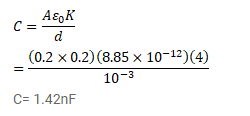# The two square faces of a rectangular dielectric slabQuestion:

The two square faces of a rectangular dielectric slab (dielectric constant 4.0) of dimensions $20 \mathrm{~cm} \times 20 \mathrm{~cm} \times 1.0 \mathrm{~mm}$ are metal-coated. Find the capacitance between the coated surfaces.

Solution: